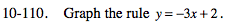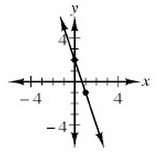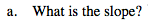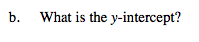### Home > MC2 > Chapter 10 > Lesson 10.2.3 > Problem10-110

10-110.Make a table of x and y-values and graph the points.Notice that the run goes in the negative x direction.

$\text{Slope} = \frac{\text{rise}}{\text{run}}$The y-intercept is the point at which the graphed line intersects the y-axis.

y = 2